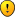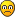## Probability Q: Guide 5- Number Properties 6th Edition Pg. 71

If you're experiencing a roadblock with one of the Manhattan Prep GMAT math strategy guides, help is here!
Pedro
Course Students

Posts: 6
Joined: Mon Mar 23, 2015 4:29 am

### Probability Q: Guide 5- Number Properties 6th Edition Pg. 71

"A bag contains equal numbers of red , green ,and yellow marbels. If Geeta pulls three marbles out of the bag, replacing each marble after she picks it, what is the probability that at least one will be red?

I understand the guide's solution:

Assuming there is one of each marble in the bag, there's a 1/3 chance of getting a red and a 2/3 chance of NOT getting a red on any given try.

P(r>=1)=1-P(r<1)=1-P(r=0)=1-(2/3)(2/3)(2/3)=1-(8/27)=19/27

What I don't understand is the more cumbersome method and why doesn't it equal 19/27?

My thinking: P(r>=1)=P(r=1)+P(r=2)+P(r=3)=(1/3)(2/3)(2/3)+(1/3)(1/3)(2/3)+(1/3)(1/3)(1/3)
= 4/27+2/27+1/27
= 7/27 (DIFFERENT than 19/27)

Can someone please tell me what I'm messing up here? Thank you in advance.RonPurewal
Students

Posts: 19746
Joined: Tue Aug 14, 2007 8:23 am

### Re: Probability Q: Guide 5- Number Properties 6th Edition Pg. 71

Pedro wrote:My thinking: P(r>=1)=P(r=1)+P(r=2)+P(r=3)=(1/3)(2/3)(2/3)+(1/3)(1/3)(2/3)+(1/3)(1/3)(1/3)
= 4/27+2/27+1/27
= 7/27 (DIFFERENT than 19/27)

here you are not accounting for the fact that there are various ways in which 'r = 1' can occur, and also various ways in which 'r = 2' can occur.
specifically, each of these can occur in three different ways. (for r = 1, the red marble could be first, second, or third. for r = 2, the NON-red marble could be first, second, or third.)

in this part, you are calculating the probability only for 'red, THEN non-red, THEN non-red'.
there are two more possibilities: 2/3 x 1/3 x 2/3 (red in the middle), and 2/3 x 2/3 x 1/3 (red at the end).
or, if you realize that all three of these possibilities are equiprobable, you can multiply what you have so far by 3.

for this part you have the same issue; there are three possibilities, of which you've accounted for just one.
so this should also be multiplied by 3.

if you make these changes you'll get an answer that agrees with the other solution.
RonPurewal
Students

Posts: 19746
Joined: Tue Aug 14, 2007 8:23 am

### Re: Probability Q: Guide 5- Number Properties 6th Edition Pg. 71

incidentally, there's an important takeaway here:
when you multiply probabilities together, you are ALWAYS taking order into account.
thus, if you multiply probabilities for things that can happen in more than one order, you MUST account for all such orders.

judging from your work, you probably weren't so clear on this. take note.
Pedro
Course Students

Posts: 6
Joined: Mon Mar 23, 2015 4:29 am

### Re: Probability Q: Guide 5- Number Properties 6th Edition Pg. 71

Thanks, Ron. That makes sense. There are 3 different permutations of the elements "(1/3)","(1/3)" and "(2/3)", similar to how many times one could arrange the world EEL.

I multiplied the first and second terms in my original equation by 3 and was able to get the correct answer.
RonPurewal
Students

Posts: 19746
Joined: Tue Aug 14, 2007 8:23 am

### Re: Probability Q: Guide 5- Number Properties 6th Edition Pg. 71

yep.
Pedro
Course Students

Posts: 6
Joined: Mon Mar 23, 2015 4:29 am

### Re: Probability Q: Guide 5- Number Properties 6th Edition Pg. 71

Ron,

Going off your logic from above, why is it that on PS #219 from OG 16, we are not multiplying (1/4)(1/2)(3/8) by the number of ways 3 distinct elements could be permuted (i.e. 6)?
RonPurewal
Students

Posts: 19746
Joined: Tue Aug 14, 2007 8:23 am

### Re: Probability Q: Guide 5- Number Properties 6th Edition Pg. 71

Pedro wrote:Ron,

Going off your logic from above, why is it that on PS #219 from OG 16, we are not multiplying (1/4)(1/2)(3/8) by the number of ways 3 distinct elements could be permuted (i.e. 6)?

in that problem, you aren't "permuting" elements, because there are NOT multiple DIFFERENT ways for the solution to turn out[/b].

here's an example:

* you have a blue die and a red die.
what's the chance that you get a 4 on the blue die, and a 5 on the red die?
this is 1/6 times 1/6.
you DON'T think about "permutations", because this CANNOT happen in multiple ways -- you have to get the 4 on the blue die, and you have to get the 5 on the red die.
you can't switch the identities of the blue and red dice, so, there are no permutations to speak of.

* you have 2 dice (not colored -- or colored the same, however you prefer to think about it).
what's the chance that you get a 4 and a 5?
this is 1/6 times 1/6 -- PLUS another 1/6 times 1/6.
one possibility is for a 4 on whichever die is designated as the "first" die, and a 5 on whichever one is designated as the "second" one. the other possibility switches those.

the problem you've cited is more like the first one of these. since the players have names, that's more like having a blue die and a red die.
KhalidA788
Course Students

Posts: 1
Joined: Thu Jun 08, 2017 10:01 am

### Re: Probability Q: Guide 5- Number Properties 6th Edition Pg. 71

Hello there,

Can someone please help me understand the P(A) way with this question... why isn't just (1/3)*(1/3)*(1/3)? isn't asking what's the probability we get a red marble on each time? so the first time the probability is 1/3, the second is 1/3, the third is 1/3? i don't know what am I missingSage Pearce-Higgins
ManhattanGMAT Staff

Posts: 943
Joined: Thu Apr 03, 2014 4:04 am

### Re: Probability Q: Guide 5- Number Properties 6th Edition Pg. 71

The question asks the probability of getting 'at least 1 red marble'. This covers lots of different scenarios: getting a red first, or second, or third, or getting two red marbles, or getting three.

The multiplication (1/3)(1/3)(1/3) describes the chance that Geeta gets three red marbles in a row, a much narrower situation than the one the question asks about.

To help with this, I'd recommend drawing a probability tree of the kind described on p109 of the NP Strategy Guide to get a sense of all the possible results in Geeta's situation.